# Lesson 17. Chart of Calculation Types, Calculation Register

Estimated duration of the lesson is 1 hour.

CONTENT:

During this lesson we will discuss configuration objects referred to as the Chart of Calculation Types and the Calculation Register, and will learn about the basic concepts employed used to create complex periodic calculations.

At the end of the lesson we will create a chart of calculation types and a calculation register which will be used during the next lesson to demonstrate the features of periodic calculations.

## Why We Need a Chart of Calculation Types and a Calculation Register

During this lesson we will discuss the features available in 1C:Enterprise to automate complex periodic calculations.

Such calculations are most commonly used to calculate wages. Therefore, we will use the payroll of employees at Jack of All Trades as an example for our discussion.

As a rule, an employee's wages are a function of many components (salary, bonuses, penalties, sick leave, nonrecurring payments, etc.). Each of these components is calculated using some algorithm that is unique to this particular component only.

For example, the amount of a penalty could be determined by a simple fixed amount, bonuses could be calculated as a percentage of salary, while salary might be calculated based on the number of working days in the month and the number of days the employee actually worked. Therefore, we will refer to each of these components as a calculation type.

An algorithm for every calculation type is generally based on two types of parameters: a period that we need to get totals for and some set of source data used in the calculation.

In reality, different calculation types generally do not exist in a vacuum, but instead somehow influence other calculations. Because of the fact that a calculation type depends on two different types of parameters, this influence is also of a twofold nature.

### Dependence by Base Period

It can influence the source data used in the calculations.

An example would be the accrual of bonuses as a percentage of salary. When salary changes, bonus also needs to be recalculated based on the new salary amount. In other words, the modified salary amount serves as the basis for calculation of bonuses.

Additionally, since the salary is calculated for a given period, when calculating bonuses we need to know not just the basic salary amount, but rather the total that is calculated for the period affecting bonus calculation. We will refer to such a period as a base period, and this dependency between calculation types will be referred to as the dependency by base period.

For example, let us discuss the accrual of bonuses for April. The bonus is going to be calculated as 10 % of the total calculated salary. So we need to analyze all the records affecting the accrual of salary for the base period we deal with, in this case for the month of April.

Suppose that the total of salary is \$8,000, in which case the bonus would come to \$800 (fig. 17.1).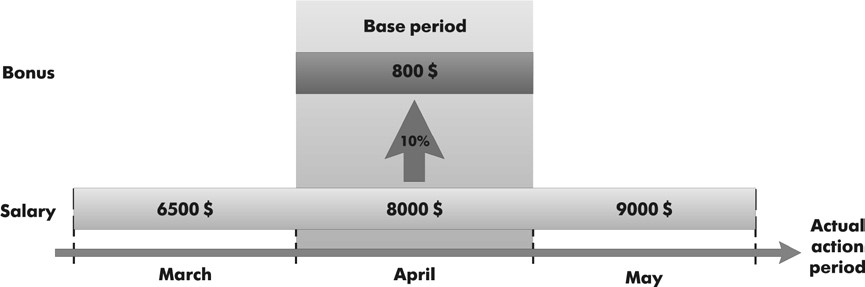Fig. 17.1. Dependency of bonuses from salary by Base Period

### Displacement by Action Period

The second type of influence is influence on the calculation period itself instead of the source data.

As an example, consider calculation for salary with absences taken into account. Suppose that we calculate an employee's salary for March. In this case the action period for the calculation is from 3/1/2010 to 3/31/2010.

Then we receive information from the departmental management that, as it turns out, the employee was absent from the 1st to the 10th of March for some unknown reason. So we will need to calculate Absence where some deductions from the employee's pay will be calculated.

But we will also need to recalculate the employee's salary based on the fact that the actual Salary calculation period is now from 3/11/2010 to 3/31/2010.

We will refer to this influence as the displacement by action period.

As a result, if the employee would have received \$9,300 for a full month's work, the accrual for the actual period will come to \$6,300 (fig. 17.2).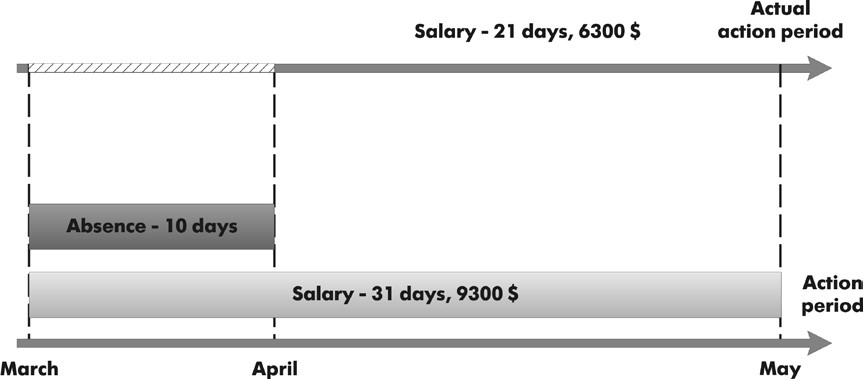Fig. 17.2. Record of Absence calculation displaces a record of Salary calculation by Action Period

Thus, based on the two types of mutual influence of calculations, one could come to a conclusion that in general there are three periods associated with every calculation type: action period, actual period, and base period.

- An action period is requested for. This means that when we specify an action period, we request as follows: "we would like the result to be valid for this period."

- Actual period is what remains after analyzing all the calculation action periods that displace portions of our calculation by action period.

- Base period is the period within which we analyze the results of other calculations that influence our calculation by that period.

As you see, the mutual influence of calculation types can vary greatly and can be multi-layered which complicates everything the most. In other words, one calculation type might influence another, which in turn affects a third, and so on.

Obviously, this situation calls for some kind of universal mechanism that will allow us to define each of these calculation types (its algorithm, influences on other calculation types, and dependencies), to provide for storage of data obtained as a result of these calculations, and to monitor the need to recalculate the results of dependant calculations upon changes in the results of "primary" calculations.

Within 1C:Enterprise this universal mechanism is achieved using chart of calculation types and calculation registers.

The first configuration object we will discuss during this lesson is the Chart of Calculation Types.

## Understanding Charts of Calculation Types

Chart of Calculation Types configuration object is intended to define information storage structures for various types of calculations. Based on a Chart of Calculation Types configuration object, the platform will create a database table to store information about calculation types available and their interrelationships.

The distinctive characteristic of chart of calculation types is that users can add new calculation types as they work. This feature makes the mechanism of periodic calculations more flexible and allows users to create their own calculation types in addition to those that are predefined by the developer.

The chart of calculation types configuration object has a property referred to as Uses Action Period. This property is used to indicate whether or not this plan contains calculation types that can be displaced by the action period.

If the property is checked, for every calculation type the developer will have the option to specify which calculation types can displace it by action period.

Another important property for Chart of Calculation Types configuration object is Dependence on Base. This property indicates whether or not this chart of calculation types contains calculation types that are dependent by base period.

If the property is checked, you will be able to indicate which chart of calculation types will contain the base calculation types and also how the dependence will be defined.

There are two possible types of base dependencies to choose from: Dependence by Action Period or Dependence by Registration Period. Both types of dependence are discussed in detail in the section titled "Understanding a Calculation Register".

Another important feature of chart of calculation types is ability to create predefined calculation types and describe their interaction. In doing so, the developer can generally define three categories of calculation types that influence the predefined types:

- Base: their results should be used upon recalculation of this type.

- Displacing: displace this calculation type by action period.

- Leading: changes in their results should force recalculation of this type.

Common sense will tell you that all the leading calculation types should encompass all the base calculation types. Besides, leading calculation types can also contain multiple other calculation types that indirectly influence this type of calculation.

For example, we have three calculation types: absence, salary, and bonus. Absence displaces salary by action period, while bonus is dependent on salary by base period.

In this situation, bonus should get salary as its base calculation type, and both salary and absence should be marked as leading, since changes to the absence calculation will force a change in the results of salary, which will then result in a change in bonus (fig. 17.3).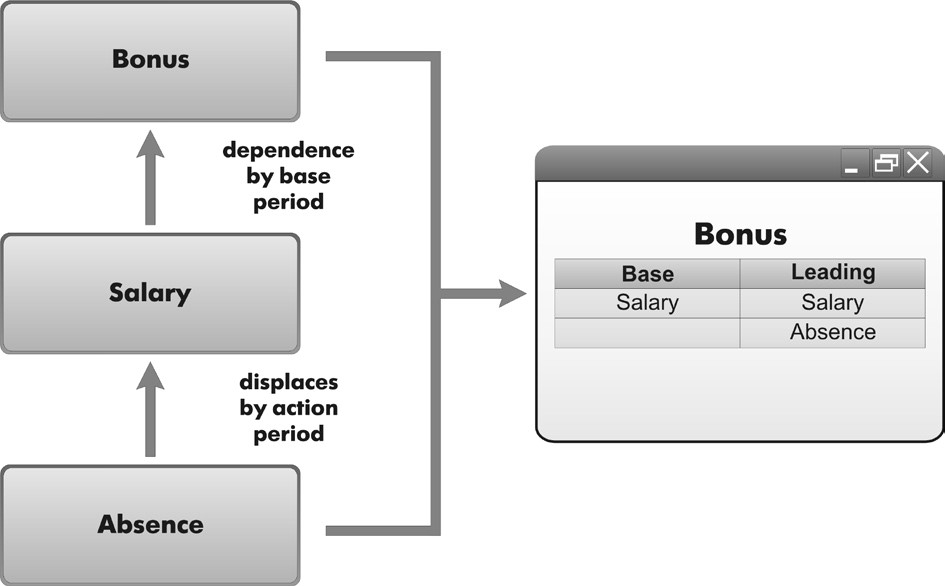Fig. 17.3. Mutual Influence of Calculation Types

For details on the structure of the 1C:Enterprise script objects intended to work with chart of calculation types, see "Developer's Quick Reference. Chart of Calculation Types".

## Adding a Chart of Calculation Types

We will now proceed to creating a chart of characteristic types named MainAccruals, which will be used in our configuration.

### In the Designer Mode

Open the Designer and create a new Chart of Calculation Types configuration object.

Enter its name as MainAccruals and also enter Calculation Types for its List Presentation.

On the Subsystems tab specify that this chart of calculation types will be displayed in the Payroll subsystem.

On the Calculation tab indicate that it will use action period and will depend on the base by action period.

We will indicate the that it will serve as its own base chart of calculation types, since all of our calculation types will be contained within a single chart of calculation types (fig. 17.4).Fig. 17.4. Defining Use of action period, Dependence on base and Base chart of calculation types

Now navigate to the Other tab and enter predefined calculation types.

As with accounting, calculations at Jack of All Trades will be rather basic, so we will only create three items (fig. 17.5):

- Absence: with Absence as the name and description, and Absence as the code;

- Salary: with Salary as its name, code and description, and set to be displaced by the Absence calculation type;

- Bonus: with Bonus as its name, code and description, and with Salary as its base calculation type and Absence and Salary as its leading calculations.Fig. 17.5. Predefined calculation types for the Main Accruals chart of calculation types

Now we will proceed to discussing the second object used in implementation of complex periodic calculations - the calculation register.

## Understanding Calculation Registers

A configuration object named Calculation Register is intended to describe the structure of accumulation for data resulting from calculations. The platform uses the Calculation Register configuration object as the basis to create database tables that will accumulate data generated by various database objects.

A calculation register is not designed to be edited directly by a user. A developer may allow users to edit a calculation register, if required.

However, the intent is for the calculation register to be modified by functions of other database objects, rather than through direct user intervention.

As with other registers, a calculation register has resources where it stores numerical data; it has dimensions, in terms of which we can get the values for the register's resources; and it has attributes which describe each calculation register record.

What makes calculation registers different is their periodicity, the ability to use the mechanisms of displacement by action period and dependence on a base period, and the connection with a chart of calculation types. We will discuss each of these features below.

### Periodicity

The periodicity of a calculation register can be set to one of the following values:

- Day;

- Month;

- Quarter;

- Year.

The calculation register periodicity determines the period of time every register record will belong to.

If the periodicity is set to Day, each record in the register will belong to some day; if periodicity is set to Month, every register record will belong to some month, etc.

To indicate the fact that a record belongs to a certain period, the register has the Registration Period system attribute of the type Date. When writing data to the register, the platform always sets the value of that attribute to the beginning of the period to which the data belongs.

For example, if data is written to a calculation register with periodicity of a month, and the RegistrationPeriod for that data is 4/8/2004, the register will store the data with the value in RegistrationPeriod field of 4/1/2004 (fig. 17.6).Fig. 17.6. Recording data from a document to a calculation register of calculation types

If in this exact situation the register periodicity is one year, the stored registration period value will be 1/1/2004 (fig. 17.7).Fig. 17.7. Recording data from a document to a calculation register of calculation types

### Displacement by Action Period

Another important feature of a calculation register is the ability to displace certain records with other records by action period.

In doing so, each calculation register record generates an actual action period which, in general, represents the sum total of several periods contained within a single action period (fig. 17.8).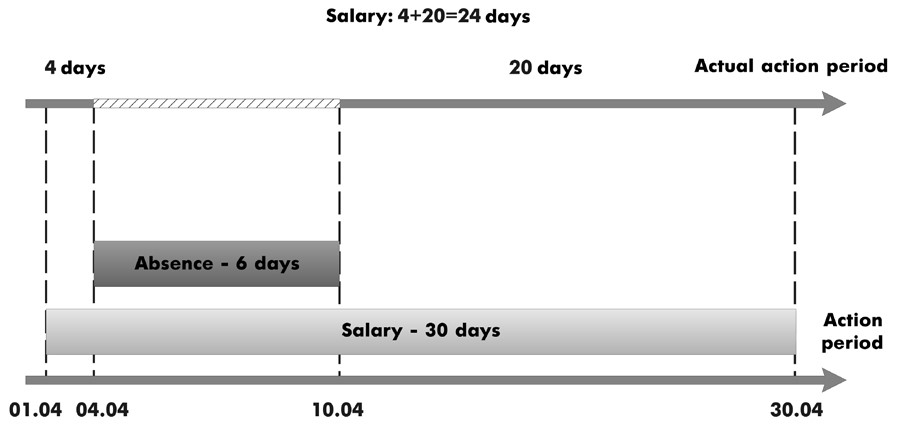Fig. 17.8. Record of Absence calculation displaces a record of Salary calculation by Action Period

If you now review the structure of records in the calculation register tables, after you enter records concerning salary calculation, register tables will look as follows (tables 17.1, 17.2):

Table 17.1. Calculation register table

 ... Start of Action period End of Action period Calculation Type ... ... ... ... ... ... ... 01.04.2004 00:00:00 30.04.2004 23:59:59 Salary ... ... ... ... ... ...

Table 17.2. Table of actual action period

 ... Start of Action period End of Action period Calculation Type ... ... ... ... ... ... ... 01.04.2004 00:00:00 30.04.2004 23:59:59 Salary ... ... ... ... ... ...

After adding records from the Absence calculation type, which displaces Salary calculations by action period, the records concerning salary calculation will look as follows (tables 17.3, 17.4):

Table 17.3. Calculation register table

 ... Start of Action period End of Action period Calculation Type ... ... ... ... ... ... ... 01.04.2004 00:00:00 30.04.2004 23:59:59 Salary ... ... 04.04.2004 00:00:00 10.04.2004 23:59:59 Absence ... ... ... ... ... ...

Table 17.2. Table of actual action period

 ... Start of Action period End of Action period Calculation Type ... ... ... ... ... ... ... 01.04.2004 00:00:00 03.04.2004 23:59:59 Salary ... ... 11.04.2004 00:00:00 31.04.2004 23:59:59 Salary ... ... ... ... ... ...

### Dependence on Base Period

Another mechanism that supports the calculation register is dependence of its records by base period. This mechanism makes it possible to base calculation of dependent (secondary) register records on the data received as the result of calculating primary records.

A calculation register can support two types of dependence on base:

dependence by action period or dependence by registration period.

#### Dependence by Action Period

Dependence by action period means that when base records are analyzed, the selected records will be those for which an intersection is found between their actual action period and the selected base period.

For example, at the beginning of April, March payroll is calculated. Bonuses for March need to be calculated based on March salaries. In this situation, as a rule, we would use dependence by action period (fig. 17.9).Fig. 17.9. Dependence by action period

There are two comments to be made regarding the figure above.

The Beginning of Base Period and End of Base Period fields are applicable only for the records with a calculation type for which dependence on the base period was specified (in our case, for calculation of bonuses).

The base value received from a specific record that has influence in the calculation, will generally not match the result contained within that record. The base will be calculated proportionally, based on what part of the influencing record's actual period overlaps with the specified base period. In the process, data of a process linked with the record will be used.

#### Dependence by Registration Period

Dependence by registration period means that when base records are analyzed, the selected records will be those with a Registration Period attribute value that falls within the specified base period.

An example is calculation of penalties when calculating salary for March. As a base for calculating the amount of penalties we will need to collect records on absences registered in March (these can include records about absences in March as well as absence records from February). In this situation, as a rule, we would use dependence by registration period (fig. 17.10).Fig. 17.10. Dependence by registration period

The final important feature of a calculation register is its connection with the chart of calculation types. It is this connection that enables displacement by action period and dependence by base period, since a chart of calculation types describes the interrelationship between calculation types.

The calculation register can have subordinate Recalculation objects. They are used to recognize the fact of records being introduced into the register, which will influence the calculation results for the register's existing records. The Recalculation configuration object can have multiple dimensions, each of which can establish a connection between the dimensions of this calculation register and those of a calculation register that influences it. In some situations this will be the same register.

Within the table created in the database based on the Recalculation object, the platform stores information regarding the register records that are subject to recalculation. The recalculation table is populated automatically, based on calculation register records that are affected by leading calculation types, as well as calculation register records for which the actual active period has changed. Using this information, a developer can decide whether register records need to be recalculated.

The last observation that we need to make in our discussion of calculation register concerns the ability to link a calculation register to a time schedule. Such a time schedule will consist of an information register (non-periodic, with a mandatory dimension of type Date, and a Number type resource), which will contain time-based source data that will take part in the calculations. The dimensions of that schedule may be, for example, a work schedule (reference to a catalog) and date, along with a resource reflecting the number of working hours for that day. In this situation it would be possible to link the calculation register to a specific work schedule (specifying a reference to a work schedule catalog as a record attribute) and then subsequently to use 1C:Enterprise script to get information about the number of working hours in the action period, actual action period or the registration period for that record.

For details on the structure of the 1C:Enterprise script objects intended to work with a calculation register, see "Developer's Quick Reference. Calculation Registers".

## Adding a Calculation Register

Before we actually create a Calculation Register configuration object named Accruals, we will first need to create two auxiliary configuration objects:

n WorkSchedules information register; n WorkScheduleTypes catalog.

We need the catalog in order to store information on the types of work schedules that are used at Jack of All Trades, and the information register will enables us to identify working days and months, since the amount of salaries will be calculated based on the number of days employees worked within the pay period.

### In the Designer Mode

Open the Designer and create a new Catalog configuration object named WorkScheduleTypes.

On the Subsystems tab specify that this catalog will be displayed in the Payroll subsystem.

On the Other tab create two predefined work schedules for the catalog (fig. 17.11): AdmininstrationSchedule and TechnicianSchedule.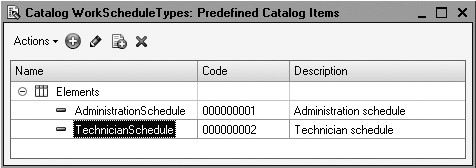Next create an Information Register configuration object named WorkSchedules.

This register will have two dimensions:

n WorkSchedule, type CatalogRef.WorkScheduleTypes; n Date, type Date.

Now create a single resource of the register: Value, with a Number type and a length of 1.

On the Subsystems tab specify that this information register will be displayed in the Payroll subsystem.

Now populate the WorkSchedules information register with the data on working days for technicians in July.

### In the 1C:Enterprise Mode

Launch 1C:Enterprise in the debugging mode and in the Payroll section use the command named Work Schedules.

Create 31 records in the register one by one.

To easier cope with this quite monotonous task, use the feature that allows adding register items by copying the current item (the button Add New Item by Copying Current (F9)). For the WorkSchedules dimension of our register select the predefined item named Technicians Schedule of the WorkScheduleTypes catalog. For the Value resource specify 1 for working days and 0 for days off (fig. 17.12).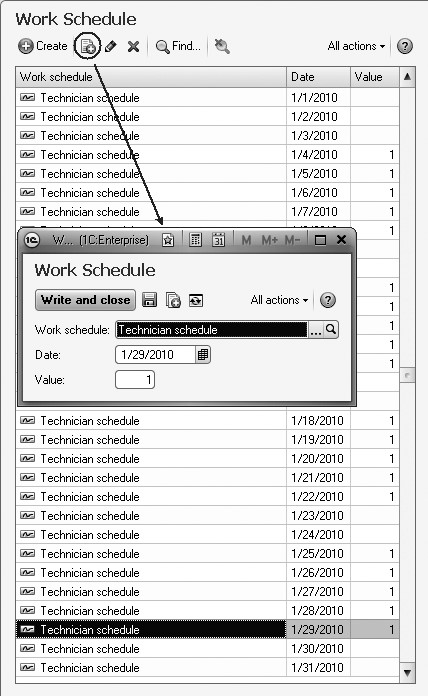Fig. 17.12. Records of the Work Schedules register

Now we are ready to create the calculation register.

### In the Designer Mode

Add a new Calculation Register configuration object named Accruals.

Select Accrual Register Records for Extended list presentation.

As a chart of calculation types for the register to use select MainAccruals.

Specify that the register will use an action period, the schedule will be provided by the WorkSchedules information register, the schedule value will be located in the Value resource, while the schedule date will be hosted in the Date dimension.

Then specify that the calculation register will use a base period and the periodicity of the register will be a Month (fig. 17.13).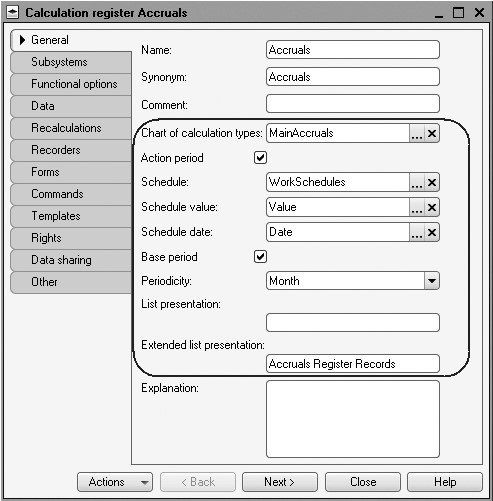Fig. 17.13. Editor of the Accruals calculation register

On the Subsystems tab specify that this calculation register will be displayed in the Payroll subsystem.

Next navigate to the Data tab and create (fig. 17.14):

- The Employee dimension, type CatalogRef.Employees, Base;

- The Result resource, type Number, a length of 15, 2 decimal places;

- The attribute WorkSchedule, type CatalogRef.WorkScheduleTypes, and in the Data property section declare Link to Schedule property by the WorkSchedule dimension;

- The SourceData attribute, type Number, a length of 15, 2 decimal places.

We will use the WorkSchedule attribute to link a register's record to a work schedule used, while the SourceData attribute will be used to store data that we may need when calculating or recalculating (in our example it will be for calculating salary).

Now navigate to the Recalculations tab.Fig. 17.14. Dimensions, resources, and attributes of a calculation register

Create a Recalculation configuration object that will be simply named Recalculation.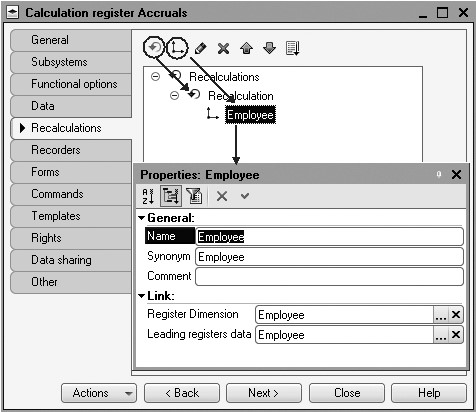Fig. 17.15. Register recalculations

It will have only one dimension - Employee. In the Link dimension for this dimension specify:

- Register dimension - Employee;

- Data of leading registers - select the same Employee dimension of the calculation register named Accruals (fig. 17.15).

Finally, edit the command interface for the command to view calculation register records to be available in the Payroll subsystem.

To do so, in the configuration object tree highlight the Payroll subsystem and select Open Command Interface from its context menu.

In the subsystem command interface window in the Navigation Panel.Normal enable visibility for the Accruals command.

This completes creation of the Accruals Calculation Register configuration object.

## Quiz

• What are complex periodic calculations?
• What is a base calculation type?
• What is the difference between the base period, actual period, and action period?
• What does dependence by base period mean?
• What is a displacement by action period?
• What is the purpose of a Chart of Calculation Types object?
• What are the major properties of a chart of calculation types?
• What is the difference between base, displacing and leading calculation types?
• How does one create a chart of calculation types?
• What is a Calculation Register configuration object?
• What are distinctive features of a calculation register?
• What is a time schedule?
• What is a recalculation?
• How are recalculation records generated?
• How does one create a calculation register?